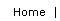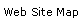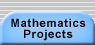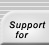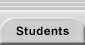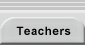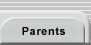Home > Mathematics Projects > Pascal’s Triangle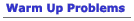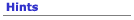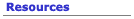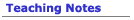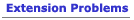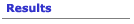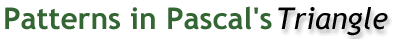How many odd numbers are in the 100th row of Pascal’s triangle? How many entries in the 100th row of Pascal’s triangle are divisible by 3? By 5? When you divide a number by 2, the remainder is 0 or 1. Color the entries in Pascal’s triangle according to this remainder. You get a beautiful visual pattern. Can you explain it? Can you generate the pattern on a computer? What about the patterns you get when you divide by other numbers? When you divide a number by 3, the remainder is 0, 1, or 2. Divide the entries in Pascal’s triangle by 3 and color them according to their remainder. Can you explain your picture?Making Mathematics Home | Mathematics Projects | Students | Teachers | Mentors | Parents | Hard Math Café | Patterns in Pascal’s Triangle Project Description | Prerequisites | Warm Up Problems | Hints | Resources | Teaching Notes | Extensions | Results |Translations of mathematical formulas for web display were created by tex4ht. © Copyright 2003 Education Development Center, Inc. (EDC)